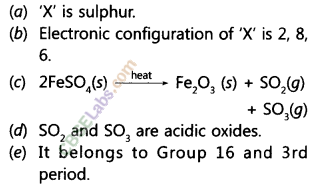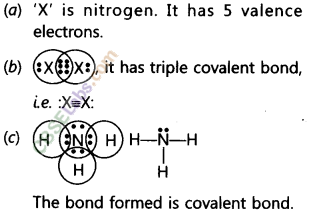# NCERT Exemplar Class 10 Science Chapter 5 Periodic Classification of Elements

NCERT Exemplar Class 10 Science Chapter 5 Periodic Classification of Elements are part of NCERT Exemplar Class 10 Science. Here we have given NCERT Exemplar Class 10 Science Chapter 5 Periodic Classification of Elements.

## NCERT Exemplar Class 10 Science Chapter 5 Periodic Classification of Elements

Question.1 “Hydrogen occupies a unique position in the Modern Periodic Table”. Justify the statement.
Answer. Hydrogen resembles with both group 1 (Alkali metals) as well as group 17 (Halogens), therefore, it occupies a unique position in the Modern Periodic Table.

Question.2 Write the formula of chlorides of Eka-silicon and Eka-aluminium, the elements predicted by Mendeleev.
Germanium is Eka-silicon. The formula isGeCl4.
Gallium is Eka-aluminium. The formula is GaCl3.

Question.3 Three elements A, B and C have 3, 4 and 2 electrons respectively in their outermost shell. Give the group number to which they belong in the Modern Periodic Table. Also, give their valenciesQuestion.4 If an element X is placed in group 14, what will be the formula and the nature of bonding of its chloride?
Answer.‘X’ belongs to group 14, it has four valence electrons and valency is equal to 4.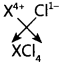The nature of bond is covalent.

(a) X has 12 protons and 12 electrons
(b) Y has 12 protons and 10 electrons
Answer. ‘X’ has 12 protons and 12 electrons. It has bigger atomic size as compared to
‘Y’ which has 12 protons and 10 electrons because effective nuclear charge is less in X than Y. Secondly, number of shells are more in ‘X’ than ‘Y’.
Therefore,
(a) ‘X’ has bigger atomic size.
(b) Y has smaller size as compared to ‘X’.

Question.6 Arrange the following elements in increasing order of their atomic radii,
(a) Li, Be, F, N
(b) Cl, At, Br, I
Answer. (a) F < N < Be < Li is increasing order of atomic size because atomic size decreases along a period from left to right.
(b) Cl < Br < I < At is increasing order of atomic size because atomic size increases down the group due to increase in number of shells.

Question.7 Identify and name the metals out of the following elements whose electronic configurations are given below.
(a) 2, 8, 2 (b) 2, 8. 1
(c) 2.8. 7 (d) 2. 1
Answer. (a) Magnesium (b) Sodium (c) Chlorine (d) Lithium
Metals are (a) Magnesium, (b) Sodium and (d) Lithium as they have 1 to 3 valence electrons. Whereas, (c) chlorine is a non-metal.

Question.8 Write the formula of the product formed when the element A (atomic number 19) combines with the element B (atomic number 17). Draw its electronic dot structure. What is the nature of the bond formed?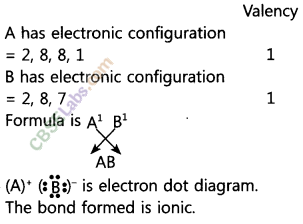Question.9 Arrange the following elements in the increasing order of their metallic character: Mg, Ca, K, Ge, Ga.
Answer. Ge < Ga < Mg < Ca < K is increasing order of metallic character. It is because ‘K’ can lose electron more easily due to larger size whereas, ‘Ge’ is smallest and cannot lose electrons.

Question.10. An element is placed in 2nd Group and 3rd Period of the Periodic Table, burns in presence of oxygen to form a basic oxide,
(a) Identify the element.
(b) Write the electronic configuration.
(c) Write the balanced equation when it burns in the presence of air.
(d) Write a balanced equation when this oxide is dissolved in water.
(e) Draw the electron dot structure for the formation of this oxide.Question.11. An element X (atomic number 17) reacts with an element Y (atomic number 20) to form a divalent halide.
(a) Where in the periodic table are elements X and Y placed?
(b) Classify X and Y as metal(s), non- metal(s) or metalloid(s).
(c) What will be the nature of oxide of element Y? Identify the nature of bonding in the compound formed.
(d) Draw the electron dot structure of the divalent halide.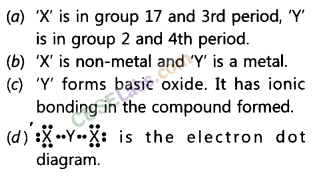(a) ‘X’ is in group 17 and 3rd period, ‘Y’ is in group 2 and 4th period.
(b) ‘X’ is non-metal and ‘Y’ is a metal.
(c) ‘Y’ forms basic oxide. It has ionic bonding in the compound formed.
(d) :X“Y”X: is the electron dot diagram.

Question.12. Atomic number of a few elements are given below.
10, 20, 7, 14
(a) Identify the elements. .
(b) Identify the Group number of these elements in the Periodic Table.
(c) Identify the Periods of these elements in the Periodic Table.
(d) What would be the electronic configuration for each of these elements?
(e) Determine the valency of these elements.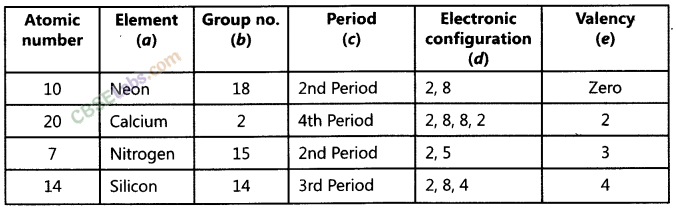Question.13 (i) Electropositive nature of the elements(s) increases down the group and decreases across the period.
(ii) Electronegativity of the element decreases down the group and increases across the period.
(iii) Atomic size increases down the group and decreases across a period (left to right).
(iv) Metallic character increases down the group and decreases across a period.
On the basis of the above trends of the Periodic Table, answer the following about the elements with atomic numbers 3 to 9.
(a) Name the most electropositive element among them.
(b) Name the most electronegative element.
(c) Name the element with smallest atomic size.
(d) Name the element which is a metalloid.
(e) Name the element which shows maximum valency.
(a) Lithium (3)
(b) Fluorine (9)
(c) Fluorine (9)
(d) Boron (5)
(e) Carbon (6). Its valency is 4.

Question.14 An element X which is a yellow solid at room temperature shows catenation and allotropy. X forms two oxides which are also formed during the thermal decomposition of ferrous sulphate crystals and are the major air pollutants.
(a) Identify the element X.
(b) Write the electronic configuration of X.
(c) Write the balanced chemical equa-tion for the thermal decomposition of ferrous sulphate crystals.
(d) What would be the nature (acidic/ basic) of oxides formed?
(e) Locate the position of the element in the Modern Periodic Table.